# RFEM – Online Manual

Online manuals, introductory examples, tutorials, and other documentation.

# 4.9 Surface Supports

Theoretical background

An elastic surface foundation represents an elastic support of all 2D elements of a surface.

In the Winkler foundation model, the soil is assumed to be an ideal liquid upon which the slab floats. This model is based on significant differences between the moduli of elasticity for concrete and (linearized) soil, which are typically 1000:1 and more. Mathematically, the assumption by Winkler is the following:

In each point, the contact pressure pz is put into relation to the displacement wz by means of the foundation constant Cz. However, the assumption implies that each point is displaced independently of all other nodes of the floor plan. Thus, the surrounding soil is irrelevant for the deformation of a surface (Figure 4.106 a).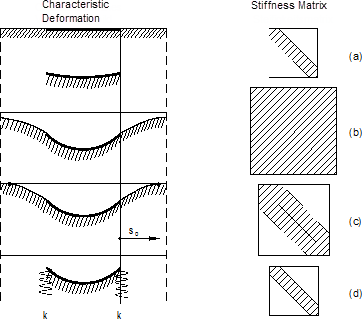Figure 4.106 Correlation between deformation figure and bandwidth of the stiffness matrix

This rudimentary foundation model is no longer up to modern requirements.

An improved type of modeling elastic foundations is based on the stiffness modulus approach: In this model, the soil is considered an elastic half-space with all its nodes correlating mathematically and mechanically. This results in an "infinitely" expanded subsidence basin, which decreases the influence of the more distant soil areas. The advantage of this mechanically superior foundation soil model, however, is offset by numerical disadvantages of a considerably larger system matrix (Figure 4.106 b).

The effective soil model according to Kolar / Nemec unifies the advantages of the two aforementioned models without having any disadvantages. This foundation soil model is based on the theory of Pasternak :

• For the slab that is in contact with the soil, only the mechanical properties of the nonlinear elastic or plastic half-space in the contact gap are important. The three-dimensional foundation effects are therefore condensed into the contact gap, i.e. transferred to a 2D problem.
• The Winkler model carries out the 2D condensation (see Equation 4.13), but is defective in terms of energy. By including a second coefficient Cv for the shear capacity of soil, the coaction of soil beyond the slab's edge is established. A natural subsidence basin with limited dimensions is formed, as it can be verified in reality.
• A two-parameter system (Cu, Cv) is created, where Cu,z approximately corresponds to the Winkler foundation constant and can be applied in this way in practical calculations. In detail, the entire system consists of five parameters: Cu,x, Cu,y, Cu,z, Cv,x, and Cv,y.

Figure 4.106 c shows this soil model in comparison. Numerically, the FE model is as stable as the Winkler model. However, incorporating the soil elements in the subsidence basin results in a larger system matrix.

The foundation soil model according to Kolar/Nemec has been enhanced as well. Experience has shown that soil elements can be eliminated from the system with the help of appropriate measures. The result is the effective soil model implemented in RFEM, symbolically shown in Figure 4.106 d. Thus, the disadvantage of the larger system matrix is eliminated. You can find a detailed description of the effective foundation soil model in .

The surrounding soil ("wedge of soil") is eliminated from the surface model by converting its rigidity into an elastic boundary line and corner node support.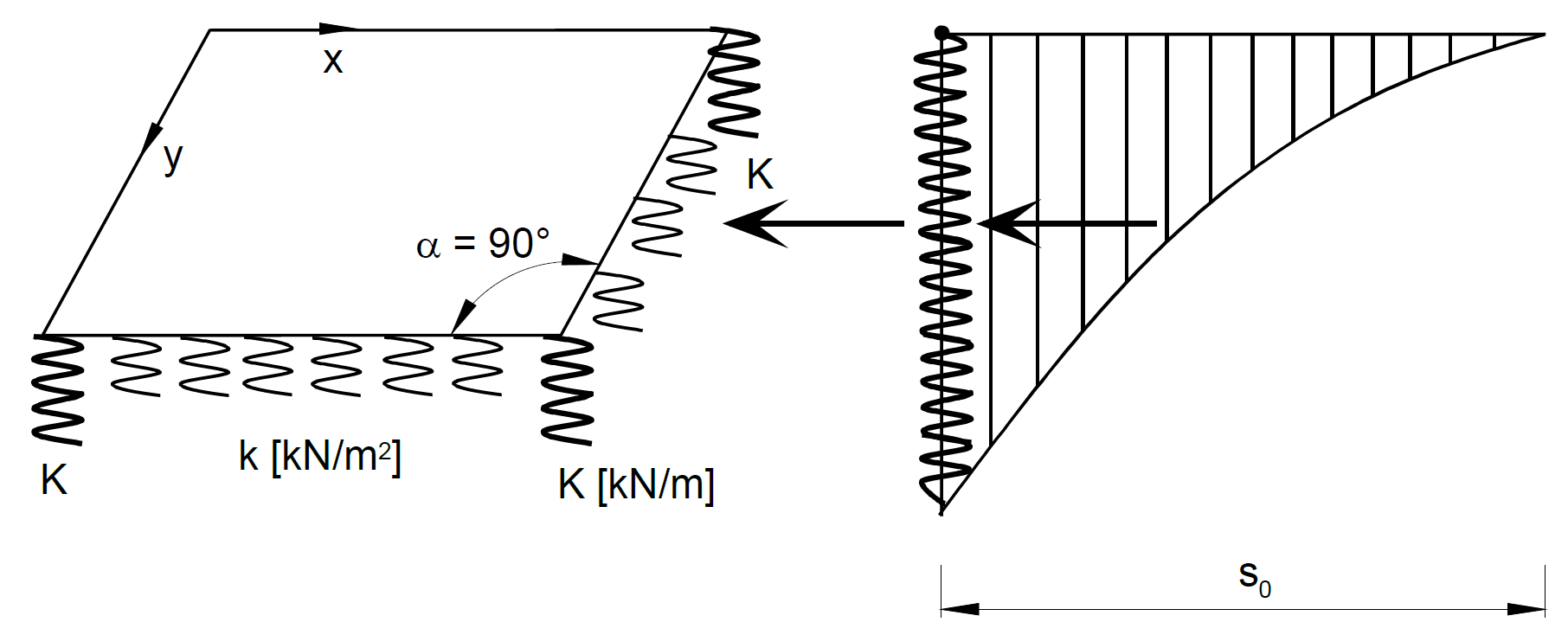Figure 4.107 Converting the surrounding soil into line and corner nodal supports

In a first approximation, the spring constants k and K of the line and corner node support are calculated according to the following equations:

Spring constant of line support:

Spring constant of corner nodal support:

In Equation 4.14, you have to insert the parameter Cv that acts perpendicular to the border line.

Equation 4.15 is used for corners with an angle α = 90° (see  for other angle sizes). Larger angles α result in smaller values K. However, if α = 0°, K = 0 as well.

The springs determined in this way must be arranged as line and nodal supports in the model in addition to the elastic foundation of the surface.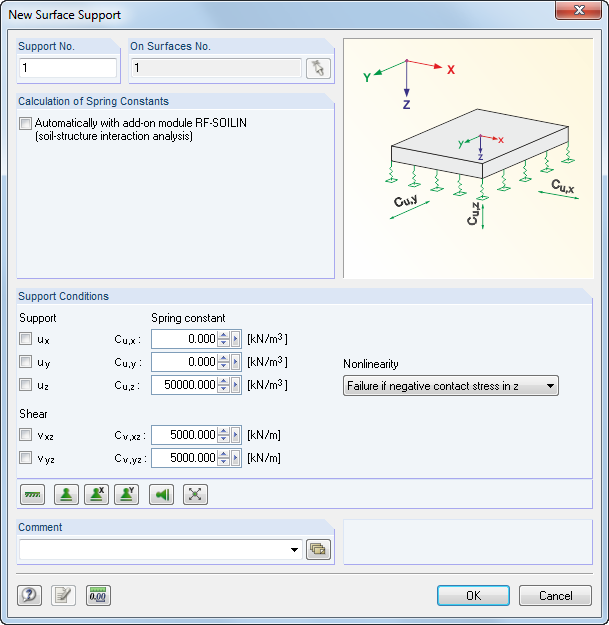Figure 4.108 New Surface Support dialog box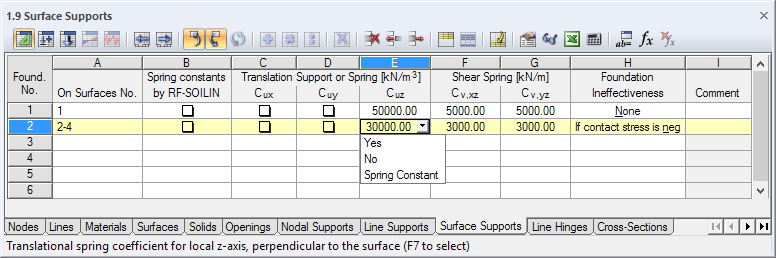Figure 4.109 Table 1.9 Surface Supports
On Surfaces No.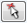Enter the numbers of the supported surfaces into this table column or text box, or select them graphically.

Spring constants by RF-SOILIN

Every soil has more or less distinctive nonlinear elastic or plastic features. To determine foundation coefficients easily, use the RF-SOILIN add-on module. The program carries out calculations of settlements, which are based on load actions and results of test borings, and determines the spring coefficients in each finite element. In the add-on module, different layers of soil can be considered at several recording points.

If the option is selected and no results of RF-SOILIN are available, the foundation coefficients are determined before the RFEM calculation is carried out.

Support or Spring Cu,x / Cu,y / Cu,z

Directions of supports or springs refer to the surfaces' local axes x, y, and z. Use the Display navigator or the shortcut menu of a surface to show them in the graphic (see Figure 4.75).

The spring stiffnesses have to be entered as design values.

If the support acts perpendicularly to the surface, enter the support or spring constant into the text box Cu,z. The parameter is practically equal to the Winkler modulus of foundation Cz. It can be taken from a soil expertise.

The parameters Cu,x and Cu,y represent translational springs that describe the foundation's resistance against displacement in the surface directions x or y. In case of a floor slab, they define the (load-independent) resistance in the horizontal directions.

In the graphic, springs are always placed in direction of the positive surface axis z. If spring symbols are on the "wrong" side of the surface, you can change the orientation of the local z-axis quickly: Right-click the surface to open the shortcut menu and select Reverse Local Axis System. This option is only available for 3D models, not for planar models. When changing settings, please note that the failure criterion changes the action direction as well.

Rigid supports make it possible to display only one part of the model, for example for symmetrical solid models. In this way, you can increase the calculation speed considerably.

Shear Spring Cv,xz / Cv,yz

These text boxes are used to consider the shear capacity of soil in direction of the surface axes x or y. In most cases, the Pasternak constant Cv lies between 0.1⋅Cu,z (minor shear capacity) and 0.5⋅Cu,z (medium shear capacity). Generally, Cv,xz = Cv,yz can be applied.

If the support uz is rigidly fixed, the corresponding shear elements for the stiffness matrix are activated automatically.

Kolar  provides a table with reference values for different soils. However, this information cannot replace the values of a soil expertise or the calculation with RF-SOILIN!

Table 4.5 Reference values for Cu,z and Cv
Soil Consistence Foundation Cu,z Shear Capacity
Cv none
Cv medium Cv high

kN/m3

kN/m

kN/m

kN/m

very soft

1,000

0

500

1,000

medium-dense

10,000

0

5,000

10,000

compact

100,000

0

50,000

100,000

The New Surface Support dialog box (see Figure 4.108) provides buttons for different support types, making the definition of degrees of freedom easier.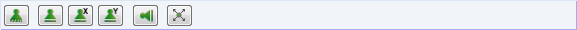Figure 4.110 Buttons in the New Surface Support dialog box

The following support properties are assigned to the buttons:

Table 4.6 Surface Support buttons
Button Support Type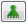Rigid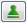Sliding in x and y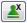Sliding in x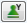Sliding in y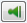Sliding in z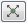Free

Nonlinearity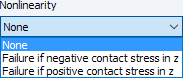The support can be excluded for positive or negative contact stresses that occur in direction of the displacement uZ: The foundation fails, for example, in case of lifting forces. Specify settings with the list in the dialog box or table (see Figure 4.109).

Positive or negative refer to the stresses acting in direction (or opposite direction) of the local z-axis of the surface: Positive contact stresses are produced when a floor slab is stressed by self-weight and the global axis Z as well as the local axis z are both oriented downwards. If the surface axis z were orientated upwards, the contact stress would be negative.

The display option of the local surface axes is shown in Figure 4.75.

Nonlinear effective surface supports are displayed in a different color in the graphic and table.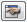Use the  button in the dialog box (see Figure 4.108) to define specific nonlinearities such as creeping (limitation of the contact stress) and friction.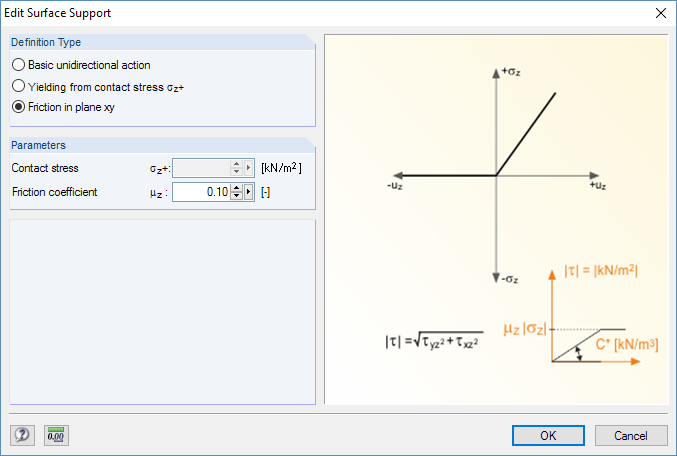Figure 4.111 Edit Surface Support dialog box

In case of a nonlinearity, RFEM calculates the deformations and stresses in several iterations. The program finds out which finite elements become stress-free if the foundation is no longer active due to failure.

For result combinations with nonlinear acting supports, there is a danger of results being combined with the locally-different support failure. In those cases, it is recommended to use load combinations (see example in Figure 5.28).

Literatur
  P. L. Pasternak. On a New Method of Analysis of an Elastic Foundation by Means of Two Foundation Constants. Gosudarstvennoe Izdatelstvo Literaturi po Stroitelstvu I Arkhitekture, Moscow, 1954. Russian.  Vladimír Kolář and Ivan Němec. Modeling of Soil-Structure Interaction. Elsevier Science Publishers with Academica Prague, Amsterdam, edition = 2. 1989.  Vladimír Kolář et al. Kurs für Statiker von Gründungsbauwerken und Erdkörpern. Haus der Technik, Ostrau, 1983. Czech.